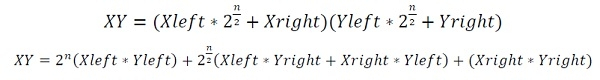# Fastest Way to multiply two Numbers

Data StructureMisc AlgorithmsAlgorithms

Two numbers are given as a binary string, our task is to find the result of multiplication for those numbers in a faster and efficient way.

Using the Divide and Conquer strategy, we can solve the problem, in a very efficient manner. We will split the numbers into two halves.

let Xleft and Xright are two parts of first number X, and Yleft, Yright are two parts of second number Y. So the product;To make it simple, we can perform this operation## Input and Output

Input:
Two binary numbers: 1101 and 0111
Output:
The result is: 91

## Algorithm

Begin
length := length of num1
carry := 0

for i := length -1 down to 0, do
num1Bit := num1[i]
num2Bit := num2[i]
sum := num1Bit XOR num2Bit XOR carry
finalSum := sum + finalSum
carry := (num1Bit AND num2Bit) OR (num2Bit AND carry) OR (num1Bit AND carry)
done

if carry ≠ 0, then
finalSum := 1 + finalSum
return finalSum
End

multiply(num1, num2)

Input: Two numbers to multiply.

Output: The result after multiplication.

Begin
length := length of num1
if n = 0, then
return 0
if n = 1, then
return (num1 * num2)
firstHalf := n/2
secondHalf := (n - firstHalf)

n1Left := substring of (0 to firstHalf) from num1
n1Right := substring of (firstHalf to secondHalf) from num1
n2Left := substring of (0 to firstHalf) from num2
n2Right := substring of (firstHalf to secondHalf) from num2

p1 := multiply(n1Left, n2Left)
p2 := multiply(n1Right, n2Right)

mask1 := shift 1 to left for 2*secondHalf bits
mask2 := shift 1 to left for secondHalf bits
End

## Example

#include<iostream>
using namespace std;

int lengthAdjust(string &num1, string &num2) {    //adjust length of binary string and send length of string
int len1 = num1.size();
int len2 = num2.size();

if (len1 < len2) {
for (int i = 0 ; i < len2 - len1 ; i++)
num1 = '0' + num1; //add 0 before the first string
} else if (len1 > len2) {
for (int i = 0 ; i < len1 - len2 ; i++)
num2 = '0' + num2; //add 0 before the second string
}
return num1.size();
}

string addBitStrings(string num1, string num2) {
string finalSum;

int length = lengthAdjust(num1, num2);    //adjust and update number lengths and store length
int carry = 0;     // Initialize carry

for (int i = length-1 ; i >= 0 ; i--) {
int num1Bit = num1[i] - '0';
int num2Bit = num2[i] - '0';

int sum = (num1Bit ^ num2Bit ^ carry)+'0';    //we know sum = A XOR B XOR C

finalSum = (char)sum + finalSum;
//the carry = (A AND B) OR (B AND C) OR (C AND A)
carry = (num1Bit&num2Bit) | (num2Bit&carry) | (num1Bit&carry);
}

if (carry)   //when carry is present
finalSum = '1' + finalSum; //add carry as MSb
return finalSum;
}

long int multiply(string num1, string num2) {
if (n == 0)    //when there is 0 length string, return 0
return 0;
if (n == 1)
return (num1 - '0')*(num2 - '0');    //perform single bit muliplication

int firstHalf = n/2;   // First half range
int secondHalf = (n-firstHalf);    // Second half range

string num1Left = num1.substr(0, firstHalf);    //first half of number 1
string num1Right = num1.substr(firstHalf, secondHalf);    //second half of number 1
string num2Left = num2.substr(0, firstHalf);
string num2Right = num2.substr(firstHalf, secondHalf);

// find left right multiplication, and multiply after adding left and right part
long int P1 = multiply(num1Left, num2Left);
long int P2 = multiply(num1Right, num2Right);

return P1*(1<<(2*secondHalf)) + (P3 - P1 - P2)*(1<<secondHalf) + P2;
}

int main() {
string num1, num2;
cout << "Enter First number in Binary: "; cin >>num1;
cout << "Enter Second number in Binary: "; cin >>num2;
cout << "The result is: " << multiply(num1, num2);
}

## Output

Enter First number in Binary: 1101
Enter Second number in Binary: 0111
The result is: 91